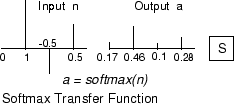# softmax

Softmax transfer function

## Syntax

``A = softmax(N)``
``info = softmax(code)``

## Description

example

``` TipTo use a softmax activation for deep learning, use `softmaxLayer` or the `dlarray` method softmax. `A = softmax(N)` takes a `S`-by-`Q` matrix of net input (column) vectors, `N`, and returns the `S`-by-`Q` matrix, `A`, of the softmax competitive function applied to each column of `N`.`softmax` is a neural transfer function. Transfer functions calculate a layer’s output from its net input.```
````info = softmax(code)` returns information about this function. For more information, see the code argument description.```

## Examples

collapse all

This example shows how to calculate and plot the softmax transfer function of an input matrix.

Create the input matrix, `n`. Then call the `softmax` function and plot the results.

```n = [0; 1; -0.5; 0.5]; a = softmax(n); subplot(2,1,1), bar(n), ylabel('n') subplot(2,1,2), bar(a), ylabel('a') ```

Assign this transfer function to layer `i` of a network.

```net.layers{i}.transferFcn = 'softmax'; ```

## Input Arguments

collapse all

Net input column vectors, specified as an `S`-by-`Q` matrix.

Information you want to retrieve from the function, specified as one of the following:

• `'name'` returns the name of this function.

• `'output'` returns the `[min max]` output range.

• `'active'` returns the `[min max]` active input range.

• `'fullderiv'` returns 1 or 0, depending on whether `dA_dN` is `S`-by-`S`-by-`Q` or `S`-by-`Q`.

• `'fpnames'` returns the names of the function parameters.

• `'fpdefaults'` returns the default function parameters.

## Output Arguments

collapse all

Output matrix, returned as an `S`-by-`Q` matrix of the softmax competitive function applied to each column of `N`.

Specific information about the function, according to the option specified in the `code` argument, returned as either a string, a vector, or a scalar.

## Algorithms

```a = softmax(n) = exp(n)/sum(exp(n)) ```

## Version History

Introduced before R2006a